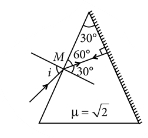Ray optics
Question

# The refractive index of the material of a prism is $\sqrt{2}$ and the angle of the prism is ${30}^{o}$ . One of the two refracting surfaces of the prism is made a mirror inwards, by silver coating. A beam of monochromatic light entering the prism from the other face will retrace its path (after reflection from the silvered surface) if its angle of incidence on the prism is

Easy
Solution

## For retracing the path shown in figure, light ray should be incident normally on the silvered face. Applying Snell’s law at point M,$\frac{\mathrm{sin}i}{\mathrm{sin}{30}^{°}}=\frac{\sqrt{2}}{1}⇒\mathrm{sin}i=\sqrt{2}×\frac{1}{2}$Get Instant Solutions